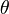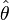# Estimator facts for kids

Kids Encyclopedia Facts

In statistics, an estimator is a rule for calculating an estimate of a given amount based on observed data: thus the rule (the estimator), the amount that is being measured (the estimand) and its result (the estimate) are different.

An estimator of a parameter$\theta$ is often written as$\hat\theta$. If the expected value of the estimator is equal to the parameter, then the estimator is called unbiased. Otherwise, it is called biased.

## Related pagesEstimator Facts for Kids. Kiddle Encyclopedia.